Chombo + EB + MF  3.2
FourthOrderMappedFineInterpSup.H File Reference

Supporting classes and routines for FourthOrderMappedFineInterp. More...

#include <cstring>
#include "NamespaceHeader.H"
#include "NamespaceFooter.H"
Include dependency graph for FourthOrderMappedFineInterpSup.H: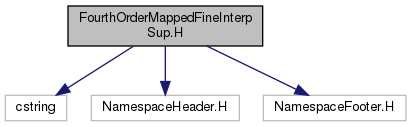Go to the source code of this file.

## Classes

class  CoordTransform
Coordinate transformations. More...

## Macros

#define _FOURTHORDERMAPPEDFINEINTERPSUP_H_

## Functions

int binomial (const int n, int k)
Calculate a binomial coefficient. More...

int powerIndex (int a_m, IntVect a_p)
Find the sequential index of a power. More...

## Detailed Description

Supporting classes and routines for FourthOrderMappedFineInterp.

## Macro Definition Documentation

 #define _FOURTHORDERMAPPEDFINEINTERPSUP_H_

## Function Documentation

 int binomial ( const int n, int k )
inline

Calculate a binomial coefficient.

Parameters
 [in] n [in] k
Returns
Binomial coefficient

References CH_assert.

Referenced by powerIndex().

 int powerIndex ( int a_m, IntVect a_p )
inline

Find the sequential index of a power.

The powers are indexed as follows (i.e for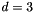dimensions)

*     int idx = 0
*     for (px = 0; px <= m; ++px)
*       for (py = 0; px+py <= m; ++py)
*         for (pz = 0; px+py+pz <= m; ++py)
*           ++idx                                                  

where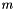is the degree of the polynomial and we wish to find idx. To compute the sequential index of any given power, we can use the relations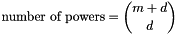and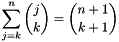With these, the amount to add to the sequential index for a power at a spatial index is total number of powers remaining at this spatial index (remainder of) minus the number of powers not used at this spatial index. E.g, if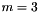,, and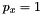, there are 2 powers left for the remaining 2 dimensions,The increment to the sequential index is then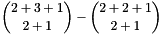In general, this can be written for direction index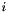, in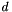space dimensions, with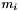giving the remaining available powers at, and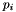giving the power used at indexas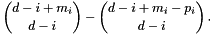Parameters
 [in] a_m Degree of the polynomial [in] a_p Power for each direction
Returns
Sequential index

References binomial(), and SpaceDim.